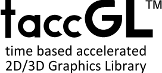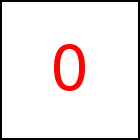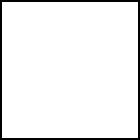# rotatePart Library Method

Short Description: Set range of rotation angle

Signature: t.rotatePart (from, to)
Group: Motion
Class: transition Class

Must be called after rotate or rotateMiddle. It defines the begin and end angle of the rotation animation. from and to are (usually floating point) numbers giving the angle sizes in radians.

The default of rotatePart(0,2*Math.PI) defines a full rotation counter clock wise. rotatePart(0,Math.PI) defines half a rotation (180 degrees). If from < to the animation runs counter clock wise. If from > to the animation runs clock wise. If from = to there is no animation at all, the object is just displayed rotated for the specified duration. from and to do not necessary have to be positive or below 2*Math.PI. So rotatePart(0,10*Math.PI) can be used to specify the object performing 5 full rotations.

### Examples

 var a=taccgl.actor("testimg"); a.rotateMiddle(0,0,1).rotatePart(0,0.5*Math.PI).start(); RUN var a=taccgl.actor("testimg"); a.rotateMiddle(1,0,0).rotatePart(0,Math.PI).start(); RUN var a=taccgl.actor("pgMiddleColumnTable"); a.rotateMiddle(0,1,0).rotatePart(0,8*Math.PI).start(); RUN var a=taccgl.actor("pgMiddleColumnTable"); a.rotateMiddle(1/Math.sqrt(2),1/Math.sqrt(2),0).dur(5).rotatePart(0,0.5*Math.PI).start(); var b=a.cont().rotateMiddle(1,0,0).rotatePart(0,1.5*Math.PI).dur(5).start(); RUN

WebGL™ is a trademark of the Khronos Group Inc.

Next Page:transition.useTM - Enable/Disable use of the Transformation Matrix
Previous Page: transition.rotateMiddle - Rotate around Objects Middle and given axis

# Motion

taccgl™ - GPU Accelerated Canvas Library